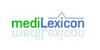### StatvoltThe statvolt is a unit of voltage and electrical potential used in the cgs system of units. The conversion to the SI system is It is a useful unit for electromagnetism because one statvolt per centimetre is equal in magnitude to one gauss. Thus, for example, an electric field of one statvolt/cm has the same energy density as a magnetic field of on...
Found on http://en.wikipedia.org/wiki/Statvolt

### statvolt(from the article `electromotive force`) ...system to one joule per coulomb of electric charge. In the electrostatic units of the centimetre–gram–second system, the unit of electromagnetic ...
Found on http://www.britannica.com/eb/a-z/s/156

### statvoltAn electrostatic unit of potential or electromotive force, equal to 299.7925 volts. ... Origin: G. Statos, standing (stationary), + volt ... (05 Mar 2000) ...
Found on http://www.encyclo.co.uk/local/20973

### StatvoltThe unit of electromotive force in the obsolete electrostatic system of units.ttle='statvolt';xiunt='statvolt';yiunt='volt';mconv=1/299.7925;cconv=0.0; See also: Stat-, Volt.
Found on http://www.diracdelta.co.uk/science/source/s/t/statvolt/source.html

### statvoltType: Term Pronunciation: stat′vōlt Definitions: 1. An electrostatic unit of potential or electromotive force, equal to 299.7925 V.
Found on http://www.medilexicon.com/medicaldictionary.php?t=84754
No exact match found